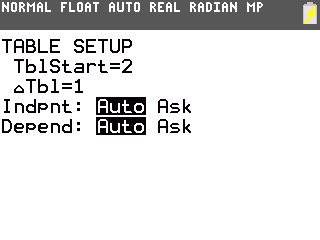# Knowledge Base

## Solution 34427: Setting the Table Modes on the TI-83 Plus and TI-84 Plus Family of Graphing Calculators.

### How can I set the different settings for the table on the TI-83 Plus and TI-84 Plus family of graphing calculators?

The instructions below show how to setup the table. In the below settings the independent variable commonly refers to the x value and the dependant variable refers to the y value as in the equation y = x + 2.

To display the TABLE SETUP screen, press [2nd][TBLSET]

TblStart (table start) defines the initial value for the independent variable. TblStart applies only when the independent variable is generated automatically, when Indpnt is set to Auto.

DTbl (table step) defines the increment for the independent variable.

Indpnt and Depend defines how the independent and dependent variables will be displayed. There are four possible settings:

1) Indpnt: Auto and Depend: Auto
Values will be displayed automatically in both the independent variable column and in all dependent variable columns.

2) Indpnt: Ask and Depend: Auto
The table will be empty. Enter a value for the independent and all the corresponding dependent variable values will be calculated and displayed automatically.

3) Indpnt: Auto and Depend: Ask
Values for the independent variable will be displayed automatically. To generate a value for a dependent variable, move the cursor to the corresponding cell in the dependent variable and press [ENTER].